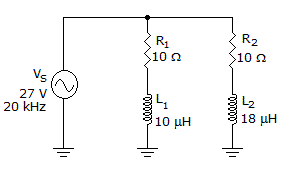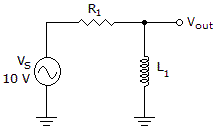# Electronics - RL Circuits

## Why Electronics RL Circuits?

In this section you can learn and practice Electronics Questions based on "RL Circuits" and improve your skills in order to face the interview, competitive examination and various entrance test (CAT, GATE, GRE, MAT, Bank Exam, Railway Exam etc.) with full confidence.

## Where can I get Electronics RL Circuits questions and answers with explanation?

IndiaBIX provides you lots of fully solved Electronics (RL Circuits) questions and answers with Explanation. Solved examples with detailed answer description, explanation are given and it would be easy to understand. All students, freshers can download Electronics RL Circuits quiz questions with answers as PDF files and eBooks.

## Where can I get Electronics RL Circuits Interview Questions and Answers (objective type, multiple choice)?

Here you can find objective type Electronics RL Circuits questions and answers for interview and entrance examination. Multiple choice and true or false type questions are also provided.

## How to solve Electronics RL Circuits problems?

You can easily solve all kind of Electronics questions based on RL Circuits by practicing the objective type exercises given below, also get shortcut methods to solve Electronics RL Circuits problems.

### Exercise :: RL Circuits - General Questions

1.

As frequency increases

 A. both series and parallel RL impedance decrease B. series RL impedance decreases and parallel RL impedance increases C. series RL impedance increases and parallel RL impedance decreases D. both series and parallel RL impedance increase

Explanation:

No answer description available for this question. Let us discuss.

2.Calculate the voltage dropped across R1 in the given circuit.

 A. 14 V B. 26.8 V C. 28 V D. 0 V

Explanation:

No answer description available for this question. Let us discuss.

3.

What is the true power of a 24 Vac parallel RL circuit when R = 45and XL = 1100?

 A. 313.45 W B. 12.8 W C. 44.96 W D. 22.3 W

Explanation:

No answer description available for this question. Let us discuss.

4.

If XL= 100and R = 100, then impedance will be

 A. 141.4B. 14.14C. 100D. 200Explanation:

No answer description available for this question. Let us discuss.

5.Which of the following statements is true if the inductor shorts out in the circuit in the given circuit?

 A. Each component drops 5 V. B. The impedance equals 0. C. The power factor equals 1. D. The phase angle equals 90°.